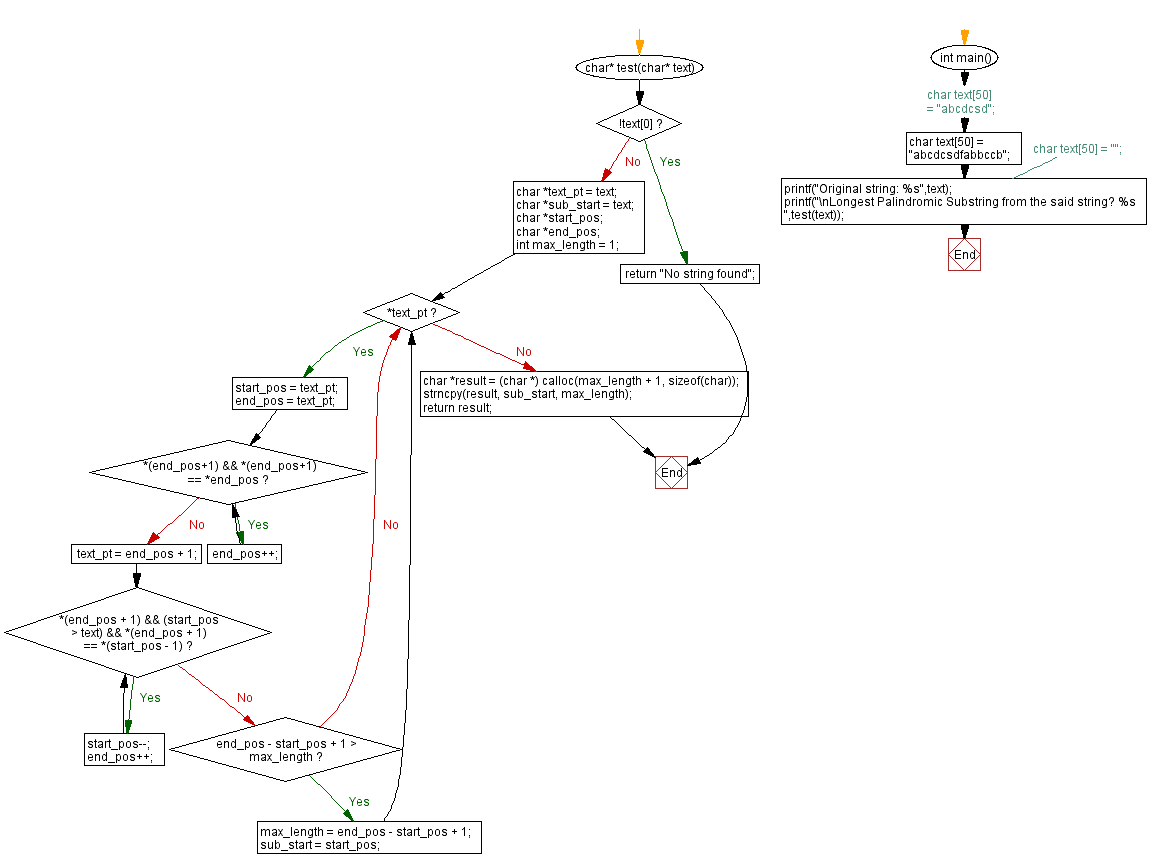﻿ C - Longest Palindromic Substring from a given string

# C Programming: Longest Palindromic Substring from a given string

## C String: Exercise-39 with Solution

From Wikipedia,
The word palindrome was introduced by Henry Peacham in 1638. A palindrome is a word, number, phrase, or other sequence of characters, which reads the same backward as forward, such as madam or racecar.

Write a C program to find the longest palindromic substring from a given string. Return the substring.

Sample Data:

("abcdcsd") -> "cdc"
("abcdcsdfabbccb") -> "bccb"
("") -> "No string found"

Sample Solution:

C Code:

``````#include <stdio.h>
#include <string.h>
#include <stdlib.h>

char* test(char* text) {
if(!text)
return "No string found";
char *text_pt = text;
char *sub_start = text;
char *start_pos;
char *end_pos;
int max_length = 1;
while(*text_pt){
start_pos = text_pt;
end_pos = text_pt;       while(*(end_pos+1) && *(end_pos+1) == *end_pos){
end_pos++;
}
text_pt = end_pos + 1;       while(*(end_pos + 1) && (start_pos > text) && *(end_pos + 1) == *(start_pos - 1)){
start_pos--;
end_pos++;
}
if(end_pos - start_pos + 1 > max_length){
max_length = end_pos - start_pos + 1;
sub_start = start_pos;
}
}   char *result = (char *) calloc(max_length + 1, sizeof(char));
strncpy(result, sub_start, max_length);
return result;
}
int main() {
//char text = "abcdcsd";
char text = "abcdcsdfabbccb";
//char text = "";
printf("Original string: %s",text);
printf("\nLongest Palindromic Substring from the said string? %s ",test(text));
}
```
```

Sample Output:

```Original string: abcdcsdfabbccb
Longest Palindromic Substring from the said string? bccb
```

Flowchart :C Programming Code Editor:

Improve this sample solution and post your code through Disqus.

Previous C Exercise: Reverse all the vowels present in a string.
Next C Exercise: Replace every lowercase letter with the same uppercase.

What is the difficulty level of this exercise?

Test your Programming skills with w3resource's quiz.

﻿